# Similarity

Are two right triangles similar to each other if the first one has a acute angle 70° and second one has acute angle 20°?

Result

#### Solution:

▵ ABC: α=70° β=20° γ=90°
▵ A'B'C': α=20° β=70° γ=90°

Leave us a comment of example and its solution (i.e. if it is still somewhat unclear...):

Showing 1 comment:Math student
Calculate the length of the third side.#### To solve this verbal math problem are needed these knowledge from mathematics:

See also our right triangle calculator. See also our trigonometric triangle calculator.

## Next similar examples:

1. Two anglesThe triangles ABC and A'B'C 'are similar. In the ABC triangle, the two angles are 25° and 65°. Explain why in the triangle A'B'C 'is the sum of two angles of 90 degrees.
2. Triangle P2Can triangle have two right angles?
3. Area of RT 2Calculate the area of right triangle whose legs have a length 5.8 cm and 5.8 cm.
4. Rectangular trapezoid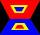How many inner right angles has a rectangular trapezoid?
5. Angles in triangle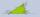Calculate the alpha angle in the triangle if beta is 61 degrees and 98 gamma degrees.
6. Triangle radians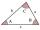The size of two internal angles of a triangle ABC are α=6/18π and β=7/18π. Calculate the size of the third angle.
7. 3-bracketMay be the largest angle in the triangle less than 20°?
8. DiagonalCan a rhombus have the same length diagonal and side?
9. Chord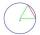Point on the circle is the end point of diameter and end point of chord length of radius. What angle between chord and diameter?
10. 3-bracket 3Two angles in a triangle are 90° and 60°. Has triangle at least two equal sides?
11. QuizQ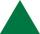An isosceles triangle has two sides of length 7 km and 39 km. How long is a third side?
12. MidpointsTriangle whose sides are midpoints of sides of triangle ABC has a perimeter 45. How long is perimeter of triangle ABC?
13. Parallelogram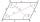In the parallelogram we know one internal angle 67°33`. Calculate the other internal angles.
14. DisjointHow many elements have union and intersection of two disjoint sets when the first have 1 and secodn 8 elements.
15. Angles 1It is true neighboring angles have not common arm?
16. Obtuse angleWhich obtuse angle is creating clocks at 17:00?
17. Rhombus anglesIf one angle in the rhombus is 159°, what is it neighboring angle in rhombus?